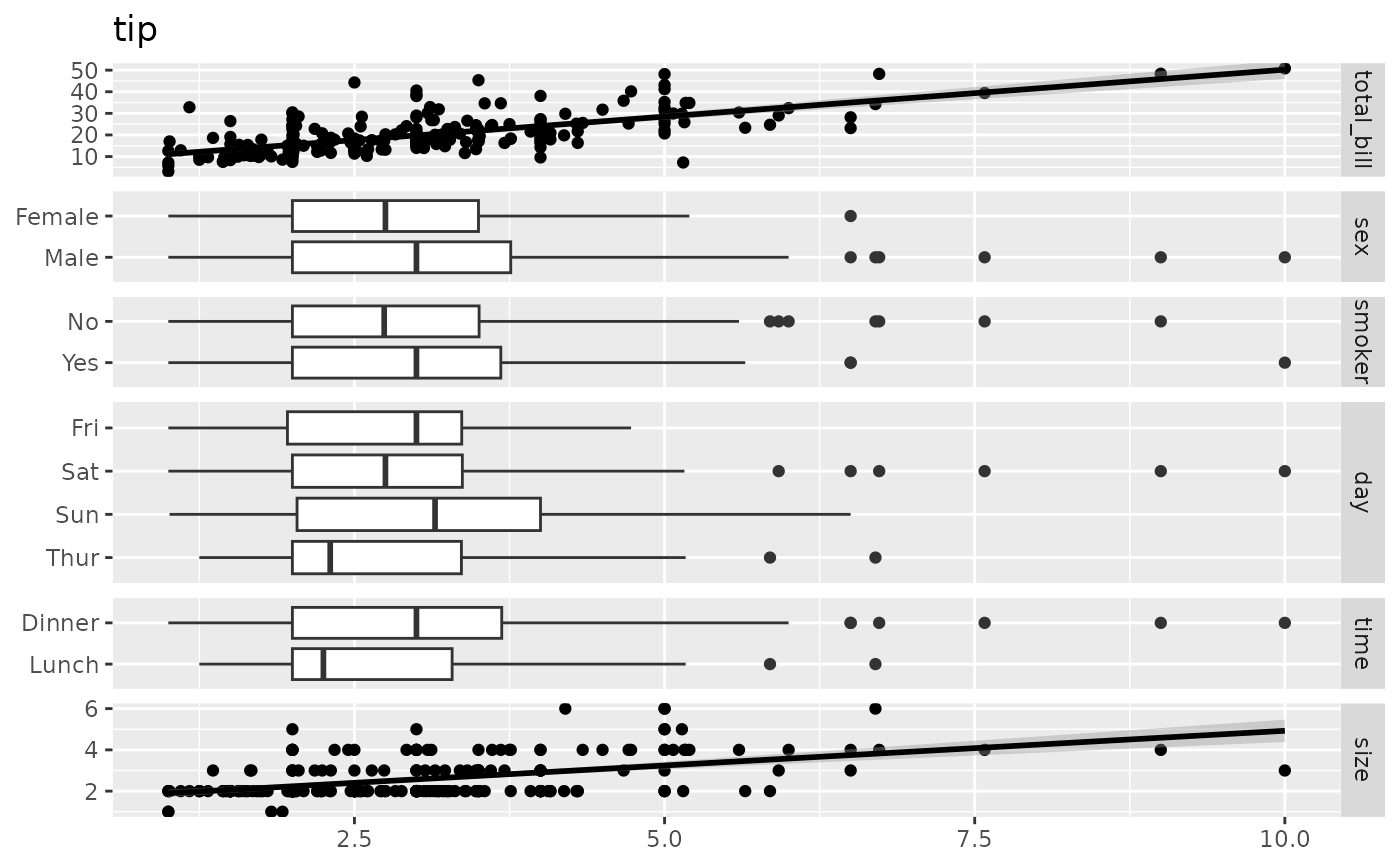ggbivariate is a variant of ggduo for plotting an outcome variable with several potential explanatory variables.

ggbivariate(
data,
outcome,
explanatory = NULL,
mapping = NULL,
types = NULL,
...,
rowbar_args = NULL
)

## Arguments

data dataset to be used, can have both categorical and numerical variables name or position of the outcome variable (one variable only) names or positions of the explanatory variables (if NULL, will take all variables other than outcome) additional aesthetic to be used, for example to indicate weights (see examples) custom types of plots to use, see ggduo additional arguments passed to ggduo (see examples) additional arguments passed to ggally_rowbar (see examples)

## Author

Joseph Larmarange

## Examples

# Small function to display plots only if it's interactive
p_ <- GGally::print_if_interactive

data(tips, package = "reshape")
p_(ggbivariate(tips, "smoker", c("day", "time", "sex", "tip")))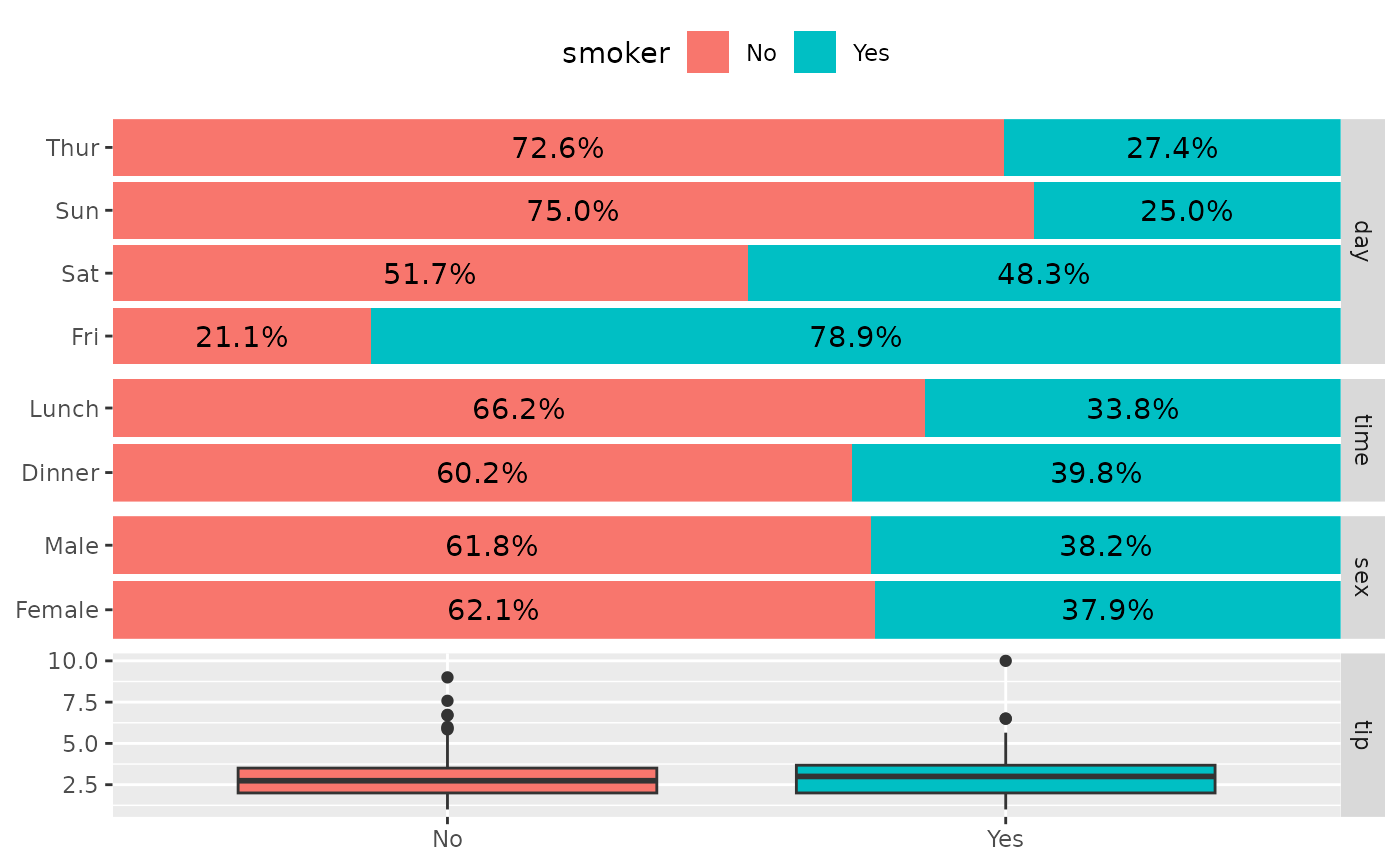# Personalize plot title and legend title
p_(ggbivariate(
tips, "smoker", c("day", "time", "sex", "tip"),
title = "Custom title"
) +
labs(fill = "Smoker ?"))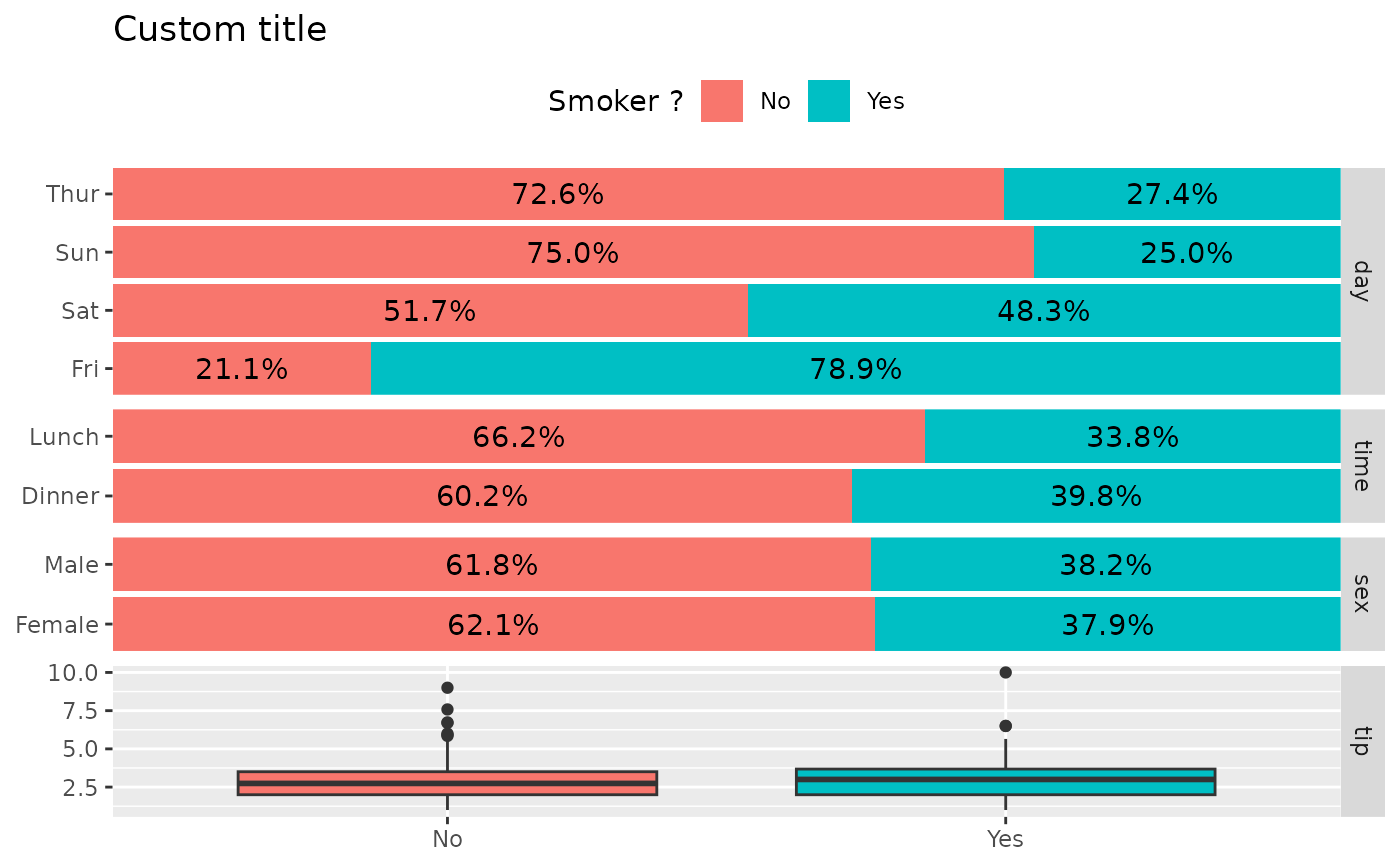# Customize fill colour scale
p_(ggbivariate(tips, "smoker", c("day", "time", "sex", "tip")) +
scale_fill_brewer(type = "qual"))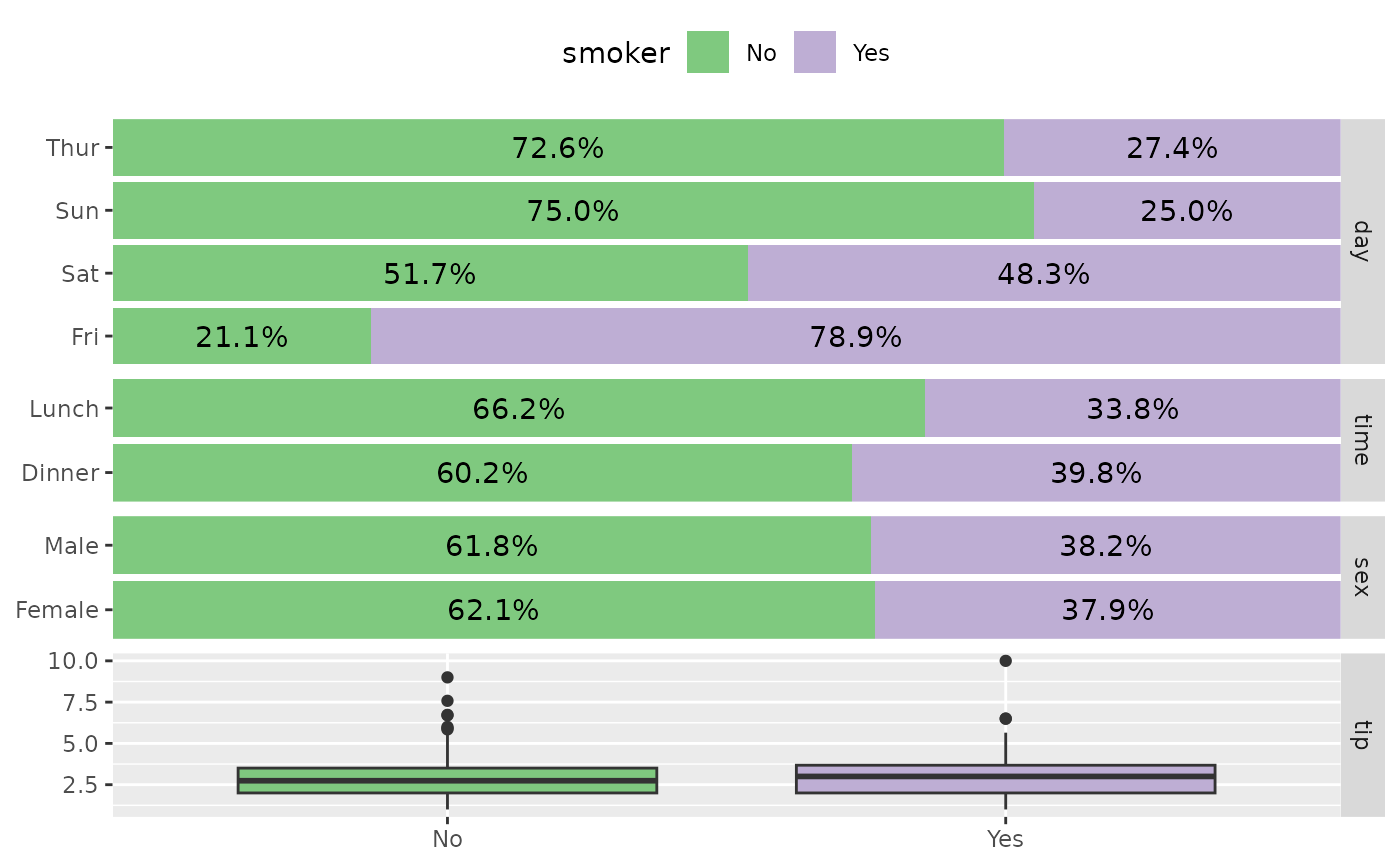# Customize labels
p_(ggbivariate(
tips, "smoker", c("day", "time", "sex", "tip"),
rowbar_args = list(
colour = "white",
size = 4,
fontface = "bold",
label_format = scales::label_percent(accurary = 1)
)
))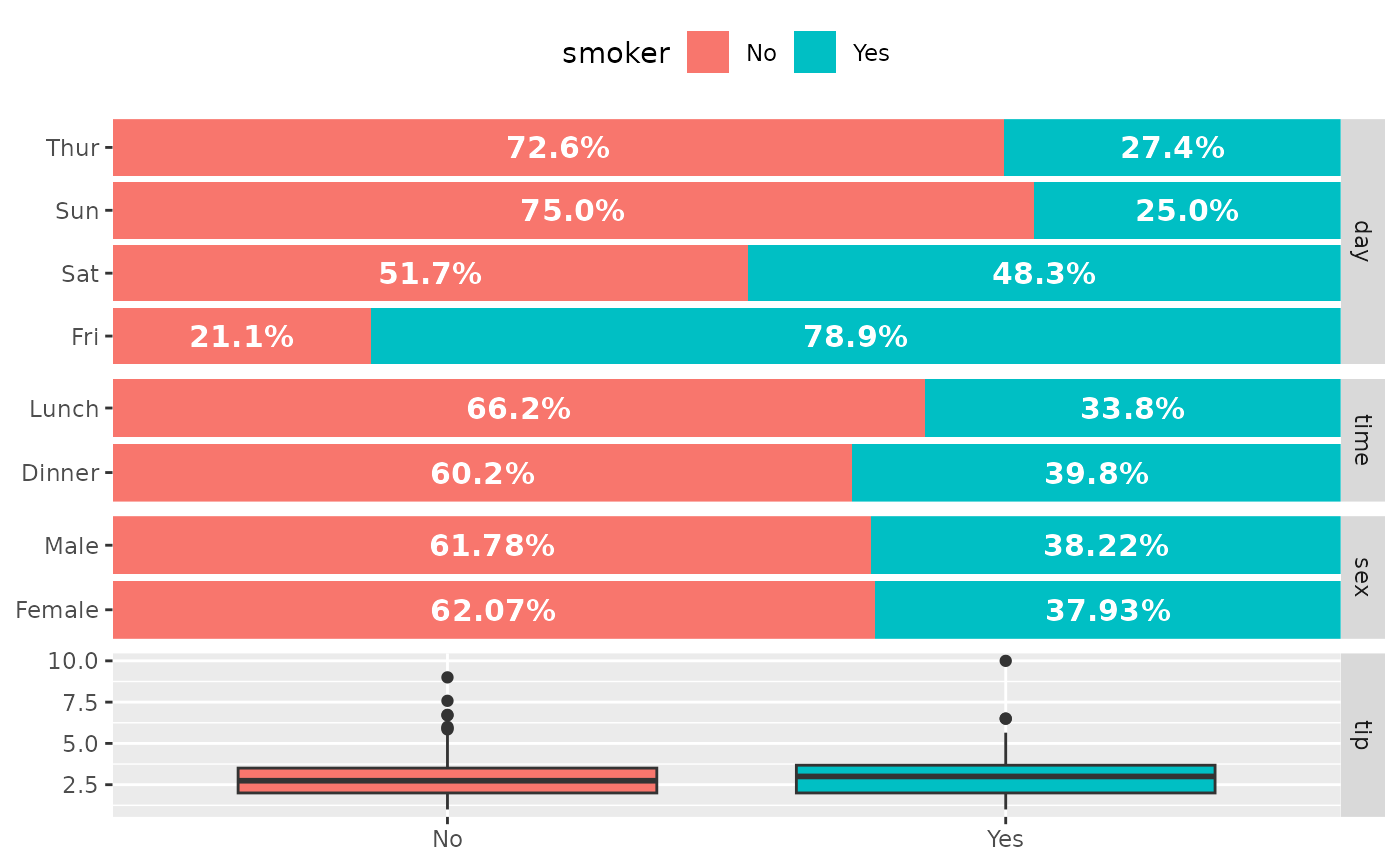# Choose the sub-plot from which get legend
p_(ggbivariate(tips, "smoker"))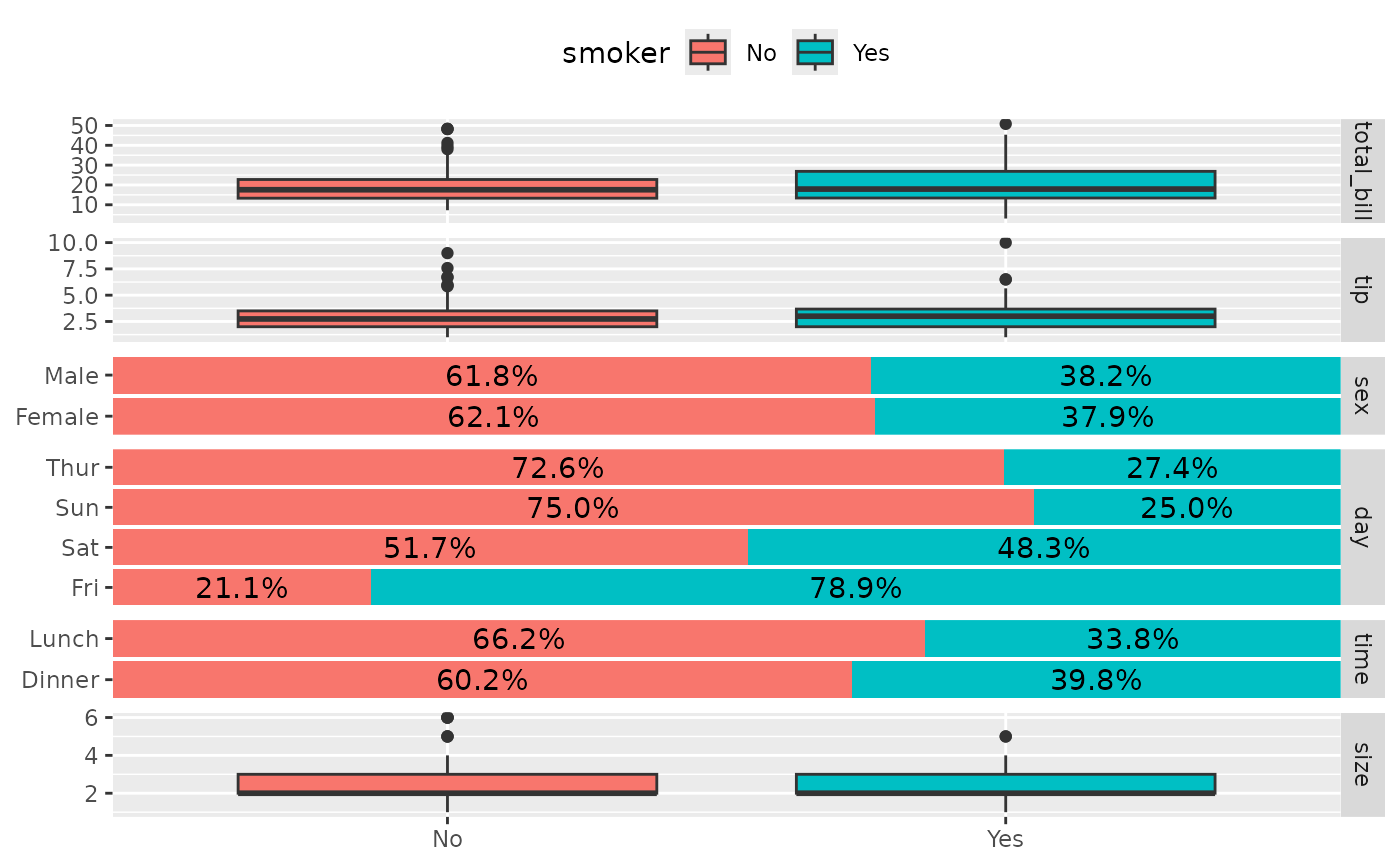p_(ggbivariate(tips, "smoker", legend = 3))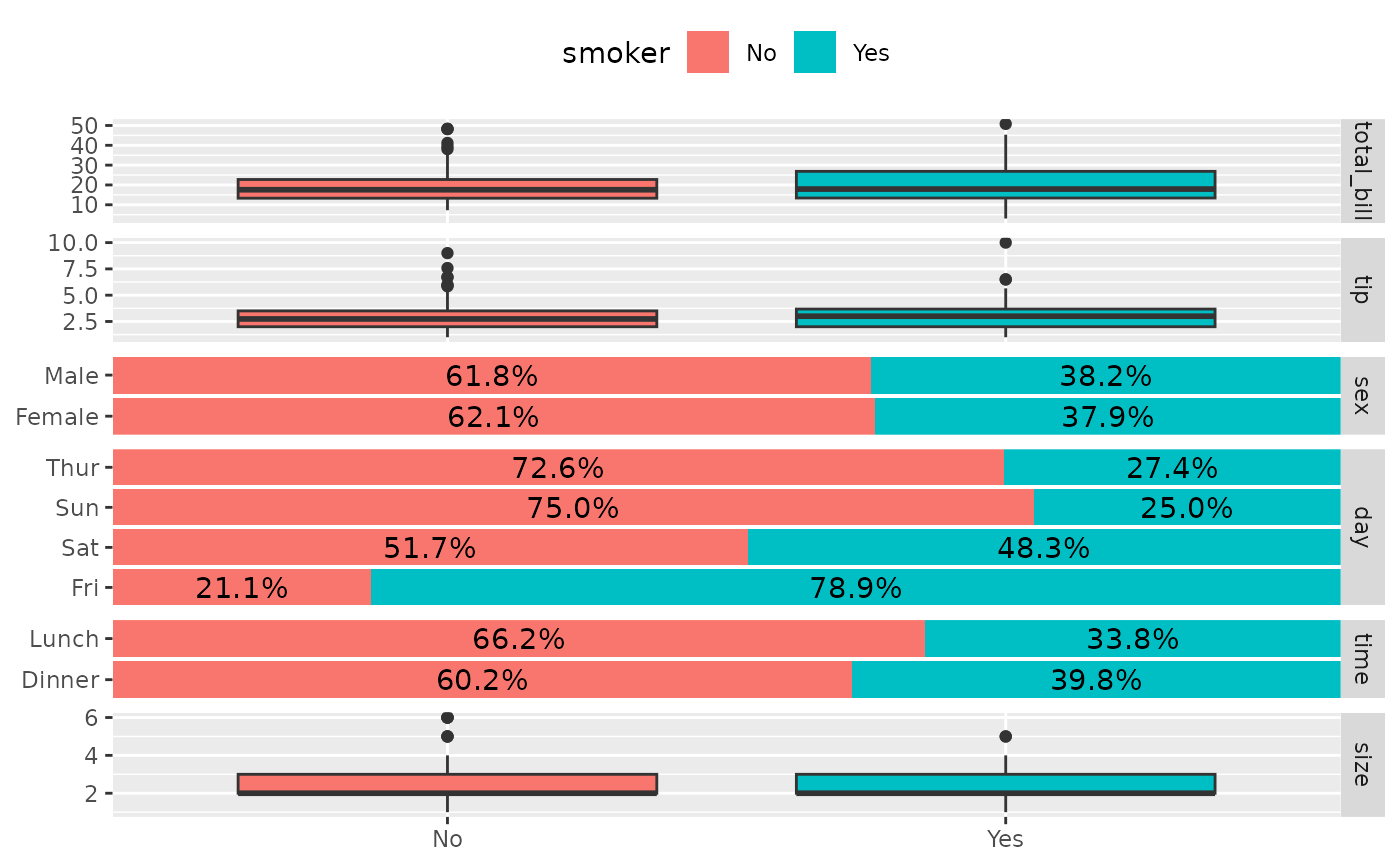# Use mapping to indicate weights
d <- as.data.frame(Titanic)
p_(ggbivariate(d, "Survived", mapping = aes(weight = Freq)))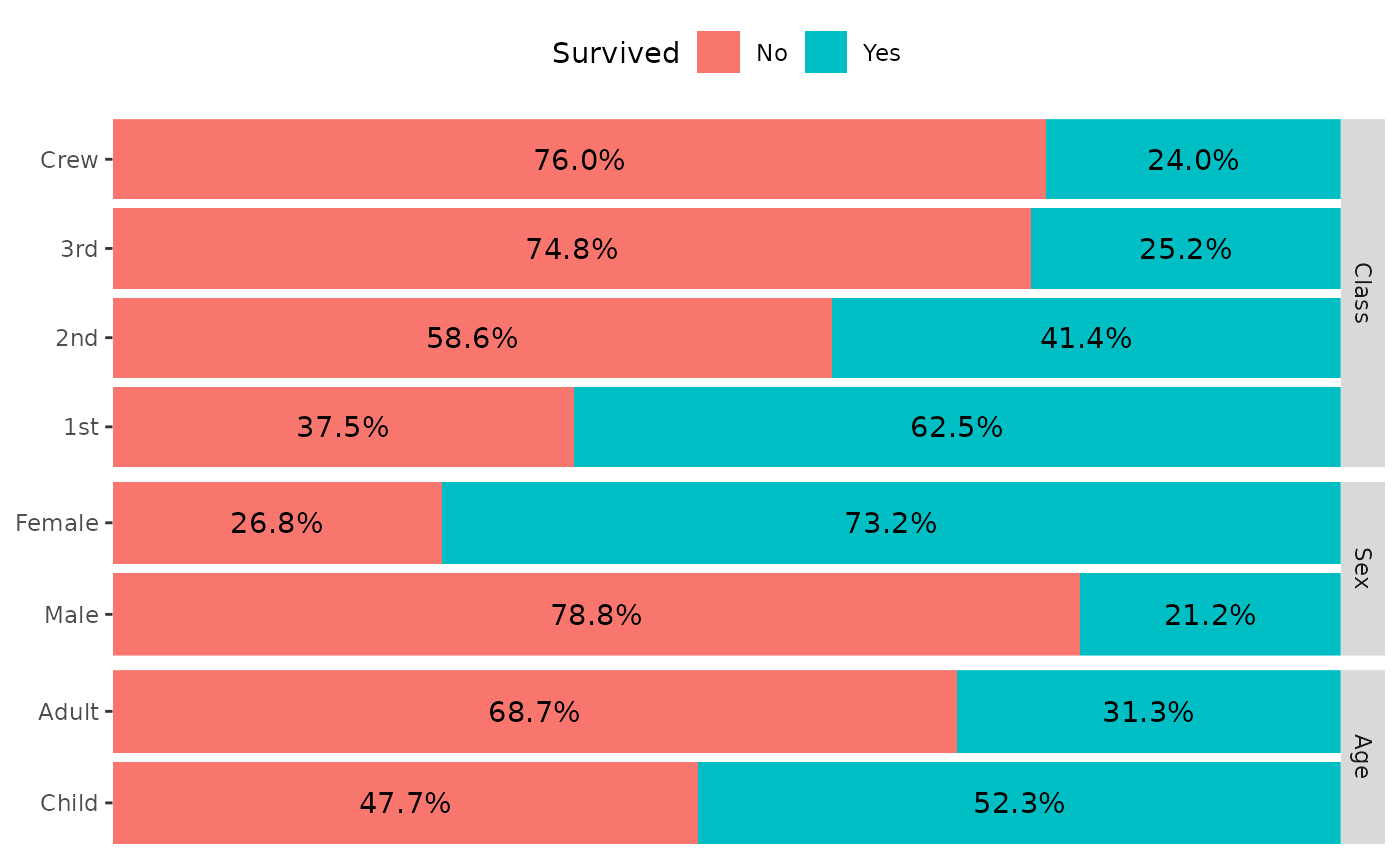# outcome can be numerical
p_(ggbivariate(tips, outcome = "tip", title = "tip"))